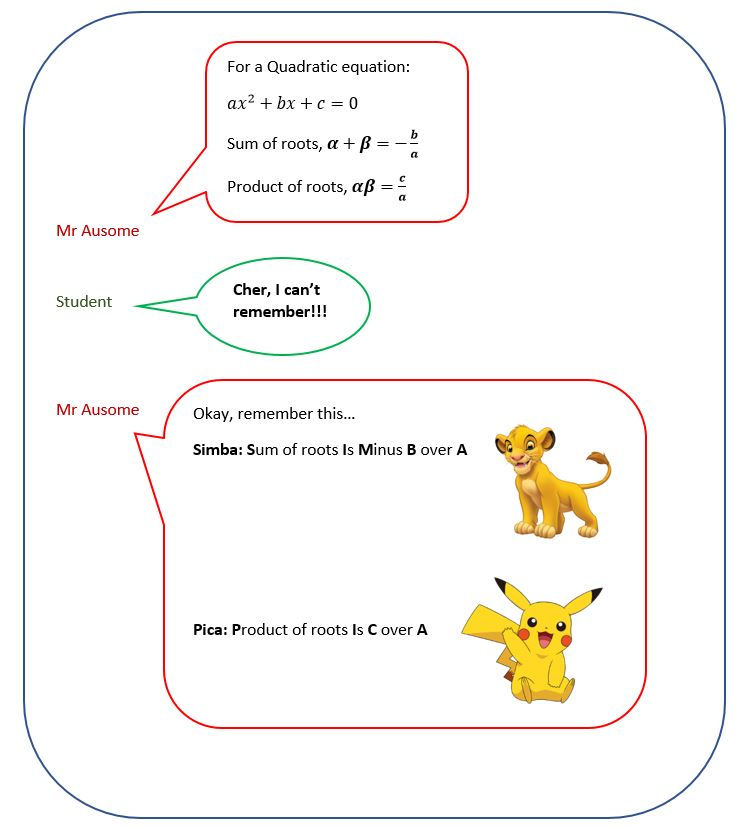top of page
• Mr Ausome

There is very little memorizing required for O-level Additional Mathematics paper.

Yet as a specialist math tutor, it is still my goal to make the few formulas as memorable as possible for my students.

The following is a cute and memorable way to remember the 2 formulas in the A-math quadratic equation chapter.Hope you found this math tip useful :)

“The average teacher explains complexity; the gifted teacher reveals simplicity.” ― Robert Brault.

Specialist Math Tutor

Tags:

bottom of page